Courses

# NCERT Exemplar Solutions: Data Handling Class 6 Notes | EduRev

## Class 6 : NCERT Exemplar Solutions: Data Handling Class 6 Notes | EduRev

The document NCERT Exemplar Solutions: Data Handling Class 6 Notes | EduRev is a part of the Class 6 Course Mathematics (Maths) Class 6.
All you need of Class 6 at this link: Class 6

Exercise Page: 72

In questions 1 to 5, out of the four options, only one is correct. write the correct answer.
Q.1. Using tally marks, which one of the following represents the number eight:
(a)(b)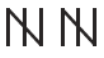(c)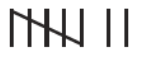(d)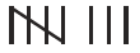Ans: (d)
Explanation: The first set of tally marks in option D represents number 5 and the second set represents number 3
So, 5 + 3 = 8

Q.2. The marks (out of 10) obtained by 28 students in a Mathematics test are listed as below:
8, 1, 2, 6, 5, 5, 5, 0, 1, 9, 7, 8, 0, 5, 8, 3, 0, 8, 10, 10, 3, 4, 8, 7, 8, 9, 2, 0
The number of students who obtained marks more than or equal to 5 is
(a) 13
(b) 15
(c) 16
(d) 17
Ans: (d)
Explanation: First we have to arrange the marks (out of 10) obtained by 28 students in a Mathematics test in an ascending order.
0, 0, 0, 0, 1, 1, 2, 2, 3, 3, 4, 5, 5, 5, 5, 6, 7, 7, 8, 8, 8, 8, 8, 8, 9, 9, 10, 10.
The number of students who obtained marks more than or equal to 5 is 17.

Q.3. In question 2 above, the number of students who scored marks less than 4 is
(a) 15
(b) 13
(c) 12
(d) 10
Solution:
Explanation: First we have to arrange the marks (out of 10) obtained by 28 students in a Mathematics test in an ascending order.
0, 0, 0, 0, 1, 1, 2, 2, 3, 3, 4, 5, 5, 5, 5, 6, 7, 7, 8, 8, 8, 8, 8, 8, 9, 9, 10, 10.
The number of students who scored marks less than 4 is 10.

Q.4. The choices of the fruits of 42 students in a class are as follows:
A, O, B, M, A, G, B, G, A, G, B, M, A, G, M, A,
B, G, M, B, A, O, M, O, G, B, O, M, G, A, A, B,
M, O, M, G, B, A, M, O, M, O,
where A, B, G, M and O stand for the fruits Apple, Banana, Grapes, Mango and Orange respectively. Which two fruits are liked by an equal number of students?
(a) A and M
(b) M and B
(c) B and O
(d) B and G
Ans: (d)
Explanation: From the question it is given that A, B, G, M and O stand for the fruits Apple, Banana, Grapes, Mango and Orange respectively.
So, by observing the choices of the fruits of 42 students in a class Banana (B) and Grapes(G) are liked by an equal number of students i.e. 8 students each.

Q.5. According to data of question 4, which fruit is liked by most of the students?
(a) O
(b) G
(c) M
(d) A
Ans: (c)
Explanation: According to data of question 4, Mango(M) is liked by most of the students.

In questions 6 to 13, state whether the given statements are true (T) or false (F).
Q.6. In a bar graph, the width of bars may be unequal.
Solution: False.
In a bar graph, bars of uniform width are drawn horizontally or vertically with equal spacing between them.

Q.7. In a bar graph, bars of uniform width are drawn vertically only.
Solution: False.
In a bar graph, bars of uniform width are drawn horizontally or vertically with equal spacing between them.

Q.8. In a bar graph, the gap between two consecutive bars may not be the same.
Solution: False.
In a bar graph, bars of uniform width are drawn horizontally or vertically with equal spacing between them.

Q.9. In a bar graph, each bar (rectangle) represents only one value of the numerical data.
Solution: True.

Q.10. To represent the population of different towns using bar graph, it is convenient to take one-unit length to represent one person.
Solution: False.
Population of towns are large numbers, then we may need a different scale.

Q.11. Pictographs and bar graphs are pictorial representations of the numerical data.
Solution: True.
By the definition, pictographs and bar graphs are pictorial representations of the numerical data.

Q.12. An observation occurring five times in a data is recorded as | | | | |, using tally marks.
Solution: False.
An observation occurring five times in a data is recorded as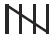using tally marks.

Q.13. In a pictograph, if a symbolrepresents 50 books in a library shelf, then the symbol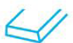represents 25 books.
Solution: True.

In questions 14 to 20, fill in the blanks to make the statements true:
Q.14. A _______ is a collection of numbers gathered to give some meaningful information.
Solution: A data is a collection of numbers gathered to give some meaningful information.

Q.15. The data can be arranged in a tabular form using _______ marks.
Solution: The data can be arranged in a tabular form using tally marks.

Q.16. A _______ represents data through pictures of objects.
Solution: A pictograph represents data through pictures of objects.

Q.17. In a bar graph, ________ can be drawn horizontally or vertically.
Solution: In a bar graph, bars can be drawn horizontally or vertically.

Q.18. In a bar graph, bars of ________ width can be drawn horizontally or vertically with ________ spacing between them.
Solution: In a bar graph, bars of uniform width can be drawn horizontally or vertically with equal spacing between them.

Q.19. An observation occurring seven times in a data is represented as ________ using tally marks.

Solution: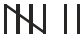An observation occurring seven times in a data is represented as using tally marks.

Q.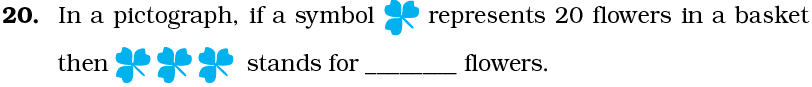Solution: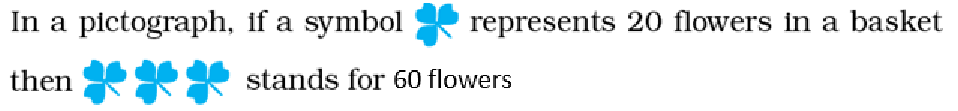From the question it is given that, one symbol represents 20 flowers.
Then, 3 symbol represents = 3 × 20
= 60

Q.21. On the scale of 1-unit length = 10 crore, the bar of length 6 units will represent ________ crore and of units will represent 75 crore.
Solution: From the question it is given that, 1 – unit length = 10 crore,
So, bar of length 6 units = 6 × 10
= 60 crore
Then, number of units will represent 75 crore = 75/10
= 7.5 units
On the scale of 1-unit length = 10 crore, the bar of length 6 units will represent 60 crore and of 7.5 units will represent 75 crore.

Q.22. In an examination, the grades achieved by 30 students of a class are given below. Arrange these grades in a table using tally marks: B, C, C, E, A, C, B, B, D, D, D, D, B, C, C, C, A, C, B, E, A, D, C, B, E, C, B, E, C, D
Solution: From the question it is given that,
The grades achieved by 30 students of a class, B, C, C, E, A, C, B, B, D, D, D, D, B, C, C, C, A, C, B, E, A, D, C, B, E, C, B, E, C, D.
These marks are represented in the table by using tally marks,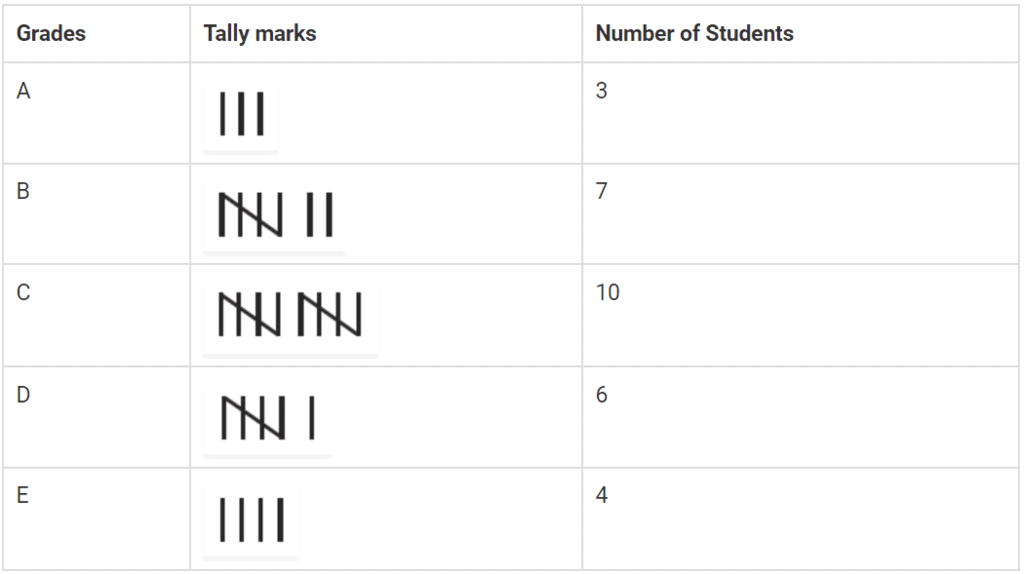Q.23. The number of two wheelers owned individually by each of 50 families are listed below. Make a table using tally marks.
1, 1, 2, 1, 1, 1, 2, 1, 2, 1, 0, 1, 1, 2, 3, 1, 2, 1, 1, 2, 1, 2, 3, 1, 0, 2, 1, 0, 2, 1, 2, 1, 2, 1, 1, 4, 1, 3, 1, 1, 2, 1, 1, 1, 1, 2, 3, 2, 1, 1
Find the number of families having two or more, two wheelers.
Solution: From the question it is given that,
The number of two wheelers owned individually by each of 50 families are,
1, 1, 2, 1, 1, 1, 2, 1, 2, 1, 0, 1, 1, 2, 3, 1, 2, 1, 1, 2, 1, 2, 3, 1, 0, 2, 1, 0, 2, 1, 2, 1, 2, 1, 1, 4, 1, 3, 1, 1, 2, 1, 1, 1, 1, 2, 3, 2, 1, 1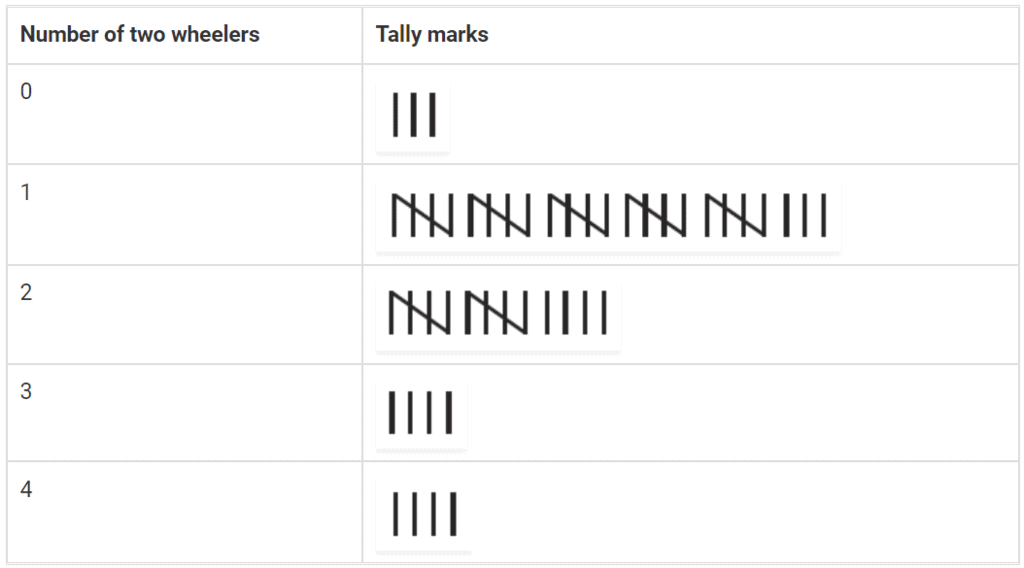Q.24. The lengths in centimeters (to the nearest centimeter) of 30 carrots are given as follows:
15, 22, 21, 20, 22,15, 15, 20, 20,15, 20, 18, 20, 22, 21,
20, 21, 18, 21, 18, 20, 18, 21, 18, 22, 20, 15, 21, 18, 20
Arrange the data given above in a table using tally marks and answer the following questions.
(a) What is the number of carrots which have length more than 20 cm?
(b) Which length of the carrots occur maximum number of times? Minimum number of times?
Solution: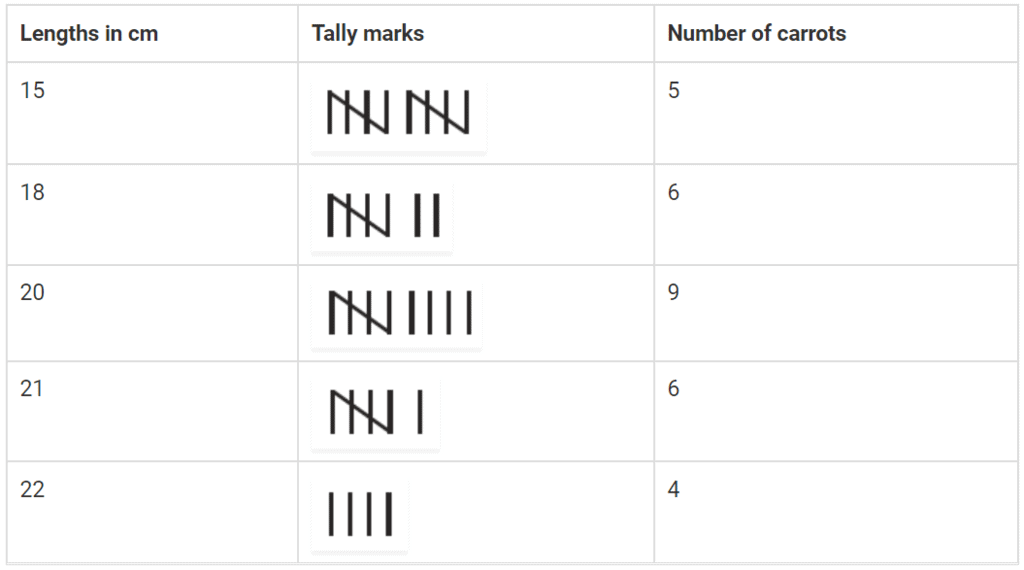(a) From the table, 10 carrots have length more than 20 cm.
(b) From the table, 20 cm length of the carrots occur maximum number of times and 22 cm length of the carrots occur minimum number of times.

Q.25. Thirty students were interviewed to find out what they want to be in future. Their responses are listed as below:
doctor, engineer, doctor, pilot, officer, doctor, engineer, doctor, pilot, officer, pilot, engineer, officer, pilot, doctor, engineer, pilot, officer, doctor, officer, doctor, pilot, engineer, doctor, pilot, officer, doctor, pilot, doctor, engineer
Arrange the data in a table using tally marks.
Solution: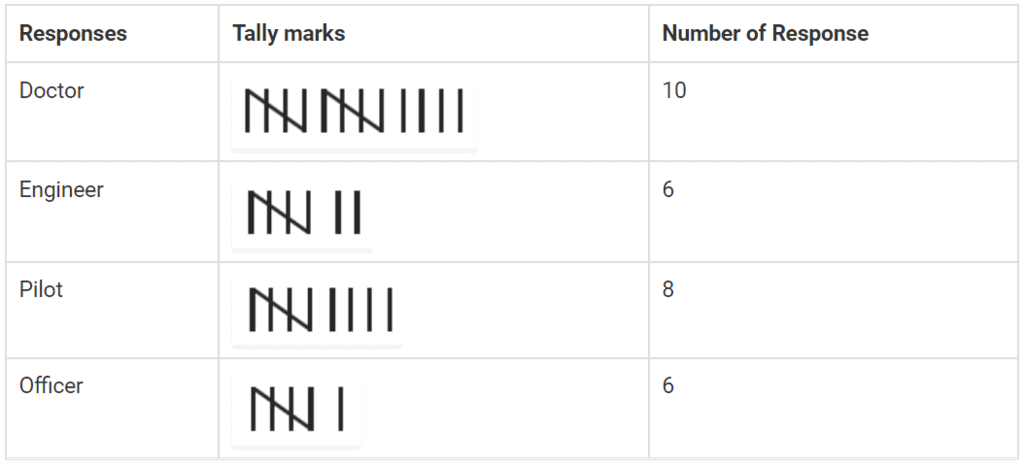Q.26. Following are the choices of games of 40 students of Class VI:
football, cricket, football, kho-kho, hockey, cricket, hockey, kho-kho, tennis, tennis, cricket, football, football, hockey, kho-kho, football, cricket, tennis, football, hockey, kho-kho, football, cricket, cricket, football, hockey, kho-kho, tennis, football, hockey, cricket, football, hockey, cricket, football, kho-kho, football, cricket, hockey, football.
(a) Arrange the choices of games in a table using tally marks.
(b) Which game is liked by most of the students?
(c) Which game is liked by minimum number of students?
Solution:
(a)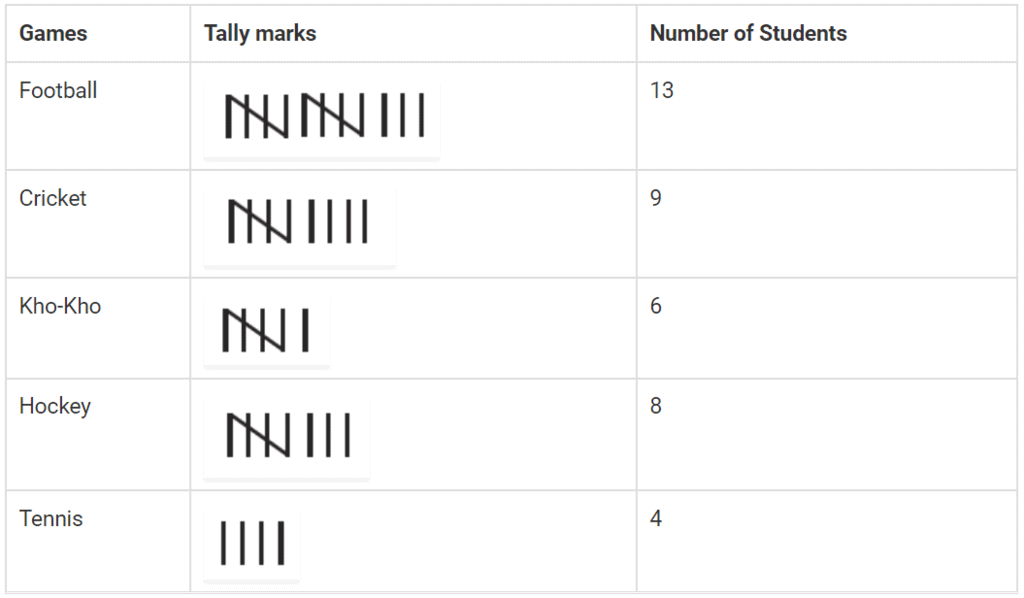(b) Football is liked by most of the students.
(c) Tennis is liked by minimum number of students.

Q.27. Fill in the blanks in the following table which represents shirt size of 40 students of a school.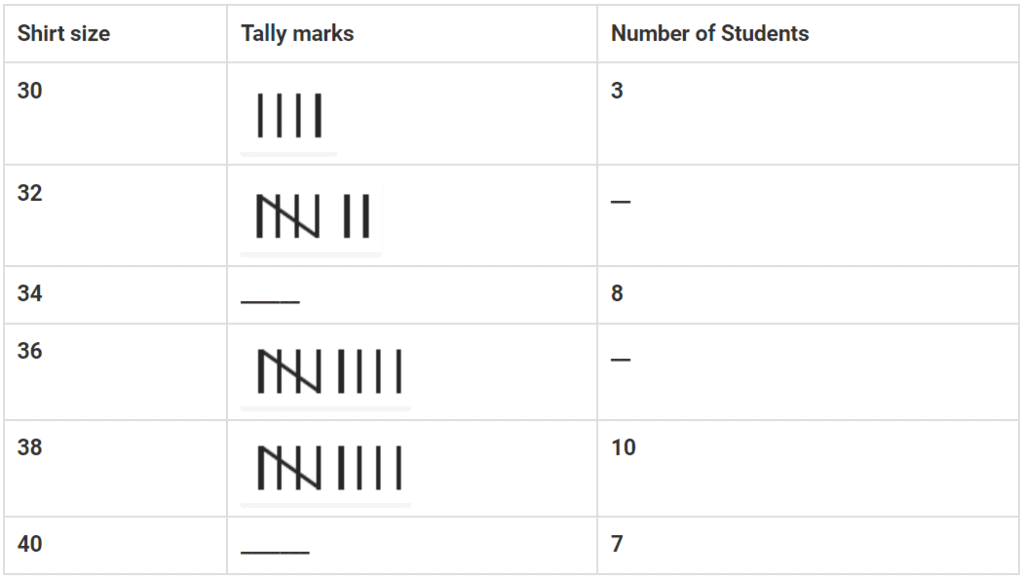Solution: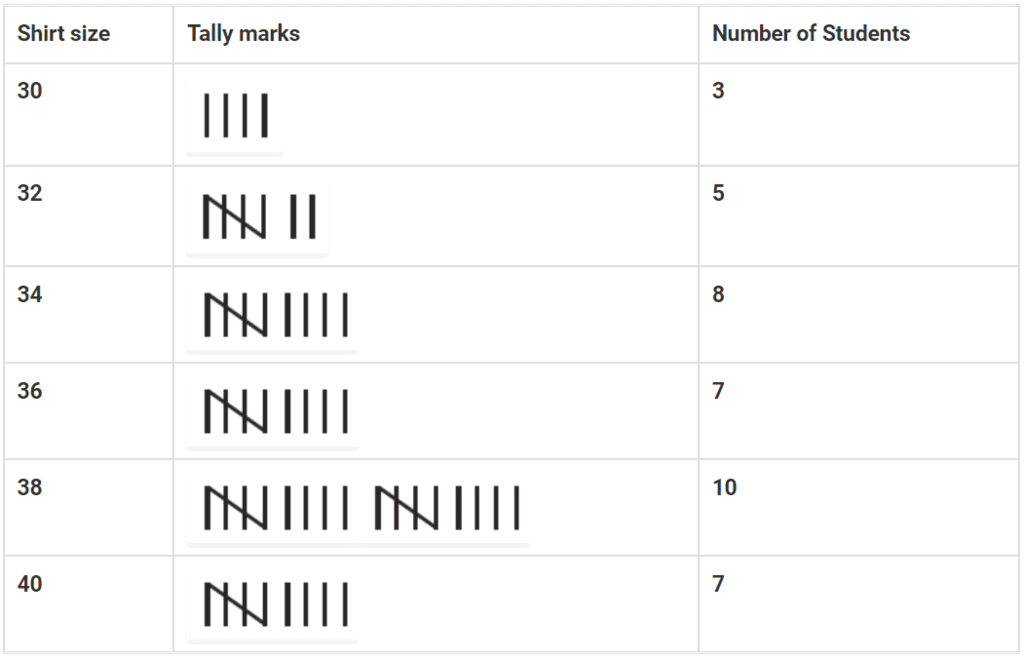Offer running on EduRev: Apply code STAYHOME200 to get INR 200 off on our premium plan EduRev Infinity!

## Mathematics (Maths) Class 6

185 videos|229 docs|43 tests

,

,

,

,

,

,

,

,

,

,

,

,

,

,

,

,

,

,

,

,

,

;